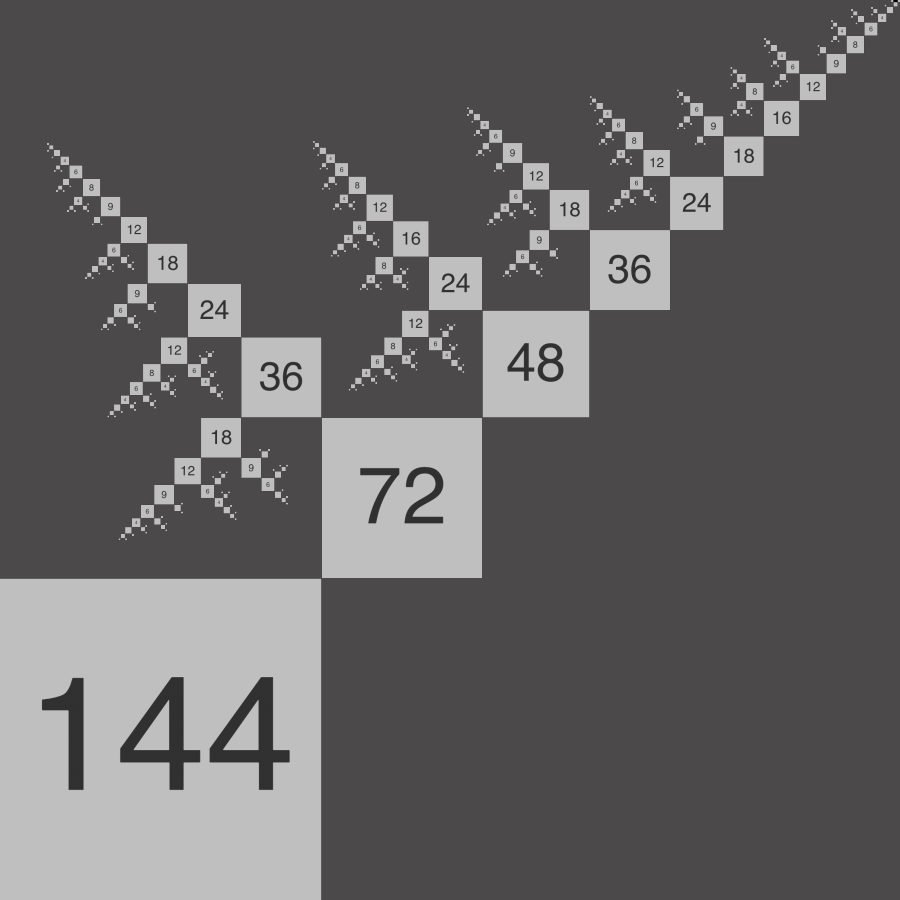# Divisor trees

A way to visualize recursively divisible numbers, a new kind of number for modular design that can be broken into parts hierarchically.

Highly divisible numbers are useful whenever a whole needs to be divided into equal parts in different ways. For example, 60 can be divided in 12 different ways. But often it is necessary to divide the parts into subparts, and subparts into sub-subparts, and so on, in a recursive way. For example, 72 can also be divided in 12 different ways but, unlike 60, the resultant subparts tend to be themselves highly divisible, as do the resultant sub-subparts, and so on. Researchers at the London Institute have discovered numbers which are divisible in this recursive way and call them recursively divisible numbers.

The extent to which a number is recursively divisible can be visualized by drawing its divisor tree.
The more squares there are in the divisor tree, the more recursively divisible the number is.
We made a web application that draws divisor trees for any given number. Divisor trees that have more squares than any of their predecessors, such as 72, 96, 120, 144 and 192, are called highly recursively composite. These numbers are especially useful for modular grid systems in graphic and digital design.